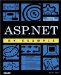# Operators and Expressions

 I l @ ve RuBoard

C# includes unary, binary, and ternary operators. Table D.2 outlines these operators.

##### Table D.2. Operator Types in C#
Category Operators
Primary (x) x.y f(x) a[x] x++ x new typeof sizeof checked unchecked
Unary + - ! ~ ++x --x (T)x
Multiplicative * / %
Shift << >>
Relational < > <= >= is
Equality == !=
Logical AND &
Logical XOR ^
Logical OR
Conditional AND &&
Conditional OR
Conditional ?:
Assignment = *= /= %= += -= <<= >>= &= ^= =

Expressions are evaluated in order of operator precedence. This precedence is based upon the associativity of the operators:

• Except for assignment operators, all binary operators are performed from left to right.

• Assignment operators and the conditional operator are evaluated from right to left.

• Precedence may be controlled through the use of parentheses.

 I l @ ve RuBoardASP.NET by Example
ISBN: 0789725622
EAN: 2147483647
Year: 2001
Pages: 154

Similar book on Amazon